Ex 14.2

Chapter 14 Class 10 Statistics
Serial order wise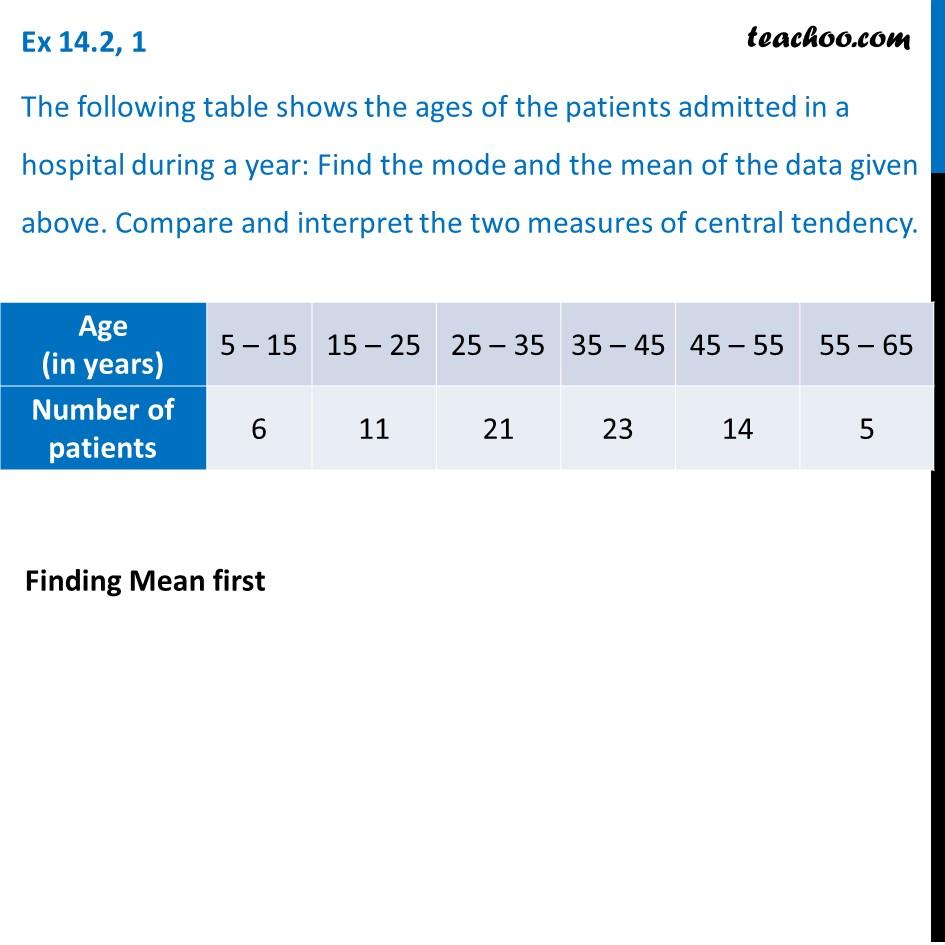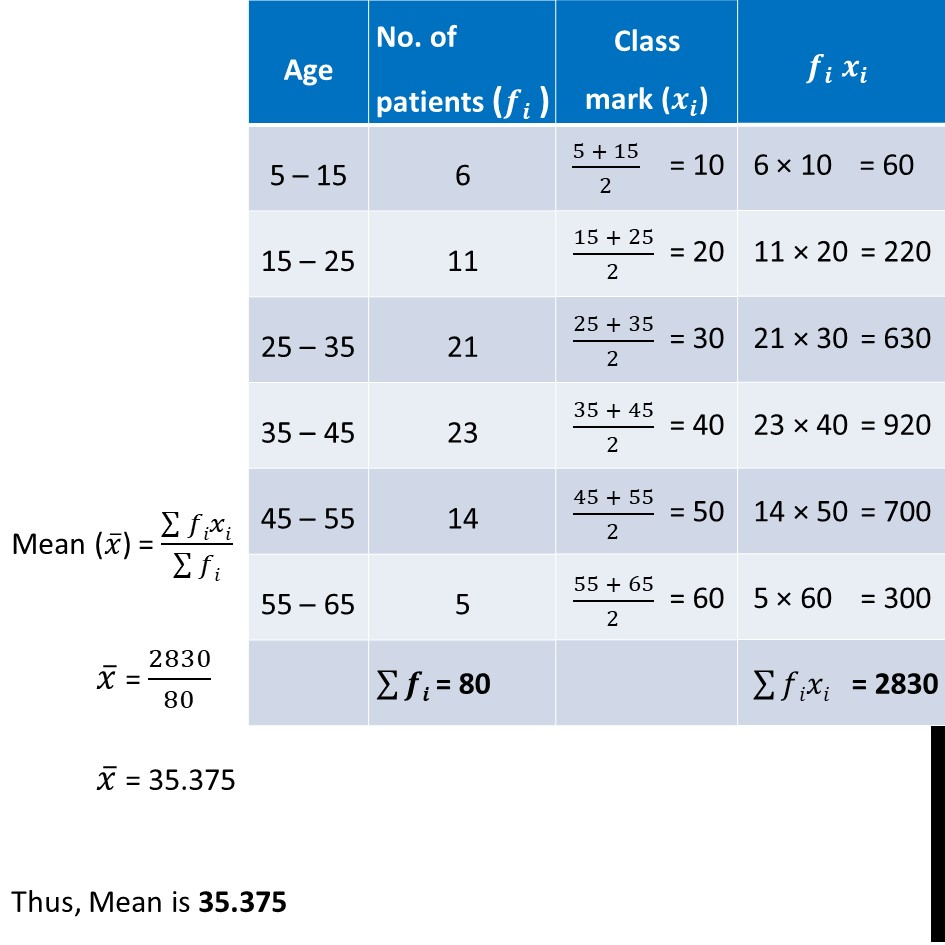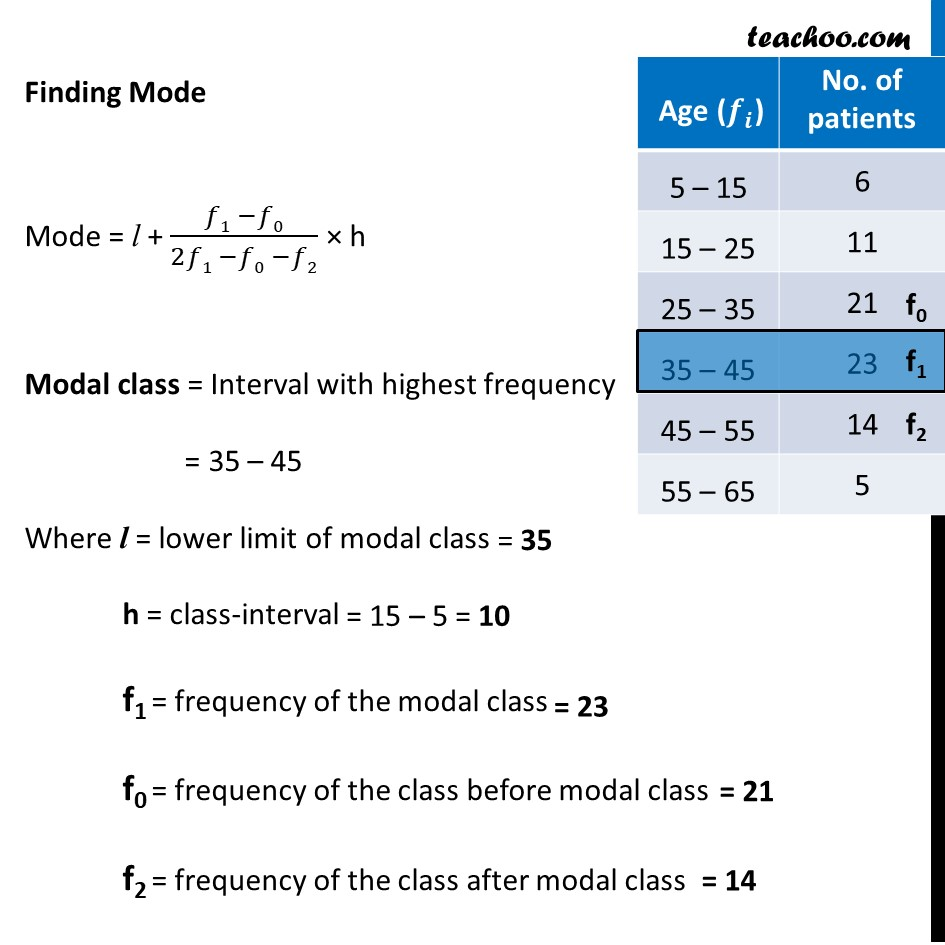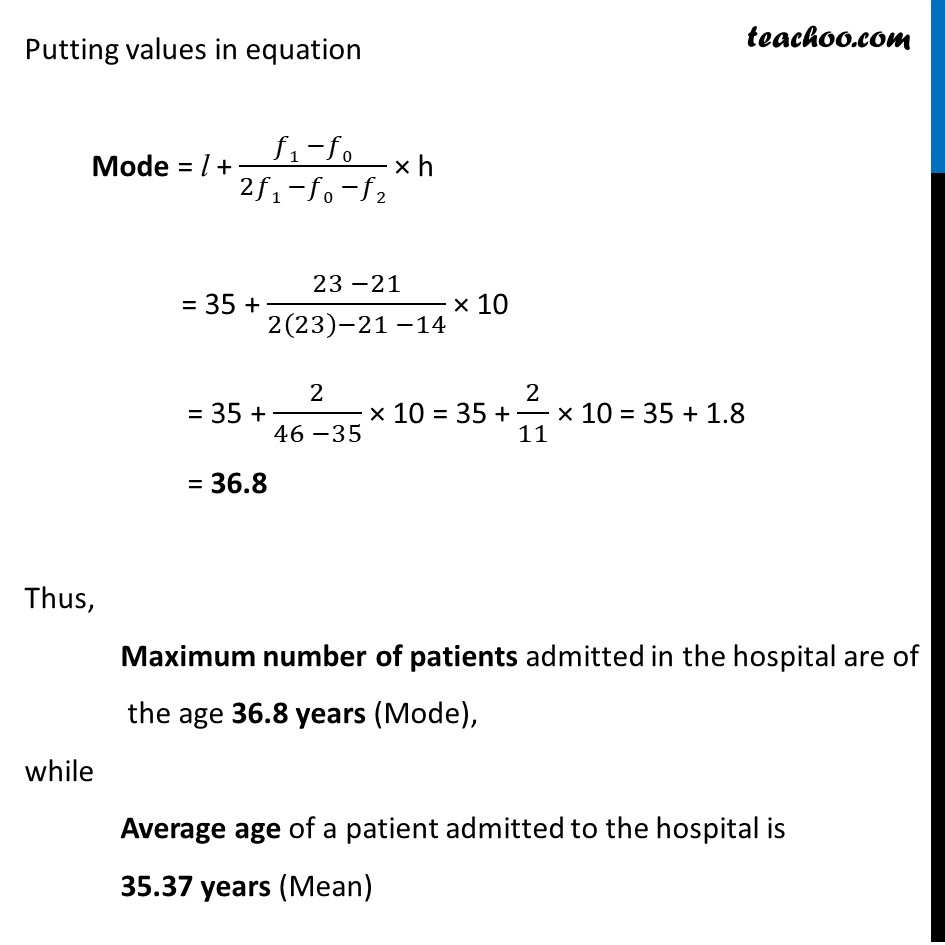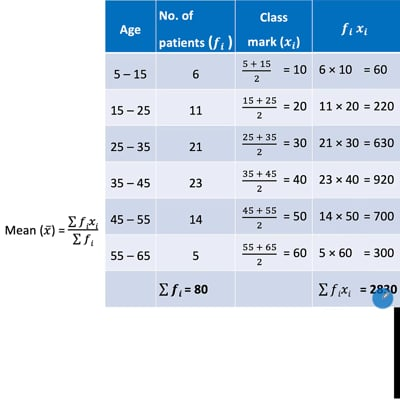This video is only available for Teachoo black users

Solve all your doubts with Teachoo Black (new monthly pack available now!)

### Transcript

Ex 14.2, 1 The following table shows the ages of the patients admitted in a hospital during a year: Find the mode and the mean of the data given above. Compare and interpret the two measures of central tendency. Finding Mean first Mean (𝑥 ̅) = (∑▒𝑓𝑖𝑥𝑖)/(∑▒𝑓𝑖) 𝑥 ̅ = 2830/80 𝑥 ̅ = 35.375 Thus, Mean is 35.375 Finding Mode Mode = l + (𝑓1 −𝑓0)/(2𝑓1 −𝑓0 −𝑓2) × h Modal class = Interval with highest frequency = 35 – 45 Where l = lower limit of modal class h = class-interval f1 = frequency of the modal class f0 = frequency of the class before modal class f2 = frequency of the class after modal class Putting values in equation Mode = l + (𝑓1 −𝑓0)/(2𝑓1 −𝑓0 −𝑓2) × h = 35 + (23 −21)/(2(23)−21 −14) × 10 = 35 + 2/(46 −35) × 10 = 35 + 2/11 × 10 = 35 + 1.8 = 36.8 Thus, Maximum number of patients admitted in the hospital are of the age 36.8 years (Mode), while Average age of a patient admitted to the hospital is 35.37 years (Mean)